# Assertion & Reason Test: Electricity - 3

## 19 Questions MCQ Test Online MCQ Tests for Class 10 | Assertion & Reason Test: Electricity - 3

Description
Attempt Assertion & Reason Test: Electricity - 3 | 19 questions in 38 minutes | Mock test for Class 10 preparation | Free important questions MCQ to study Online MCQ Tests for Class 10 for Class 10 Exam | Download free PDF with solutions
QUESTION: 1

### Directions: In the following questions, a statement of assertion (A) is followed by a statement of reason (R). Mark the correct choice as:Assertion : The connecting wires are made of copper.Reason : The electrical conductivity of copper is high.

Solution: Copper conducts the current without offering much resistance due to high electrical conductivity.
QUESTION: 2

### Directions: In the following questions, a statement of assertion (A) is followed by a statement of reason (R). Mark the correct choice as:Assertion : A conductor has +3.2 X 10-19C charge.Reason : Conductor has gained 2 electrons.

Solution: Conductor has positive charge, so it has lost two electrons.
QUESTION: 3

### Directions: In the following questions, a statement of assertion (A) is followed by a statement of reason (R). Mark the correct choice as:Assertion : If p1 and p2 be the resistivity of the materials of two resistors of resistances R1 and R2 respectively and R1 > R2Reason : The resistance R = pl/A ⇒ p1> p2 if R1 > R2

Solution: p is the characteristic of. the material of resistors. It does not depend on the length and cross-sectional area of resistors. But R depends on the length and the cross-sectional area of the resistor. So, R1 may be greater than R2 even when pi ≤ p 2.
QUESTION: 4

Directions: In the following questions, a statement of assertion (A) is followed by a statement of reason (R). Mark the correct choice as:

Assertion : Wire A is thin in comparison to wire B of same material same length then resistance of wire A is greater than resistance of wire B.

Reason: Resistivity of wire A is greater than resistance of wire B.

Solution: Resistivity is a material property. And Resistance is inversely proportional to the cross section area of the wire. So, thinner the wire, higher the resistance.
QUESTION: 5

Directions: In the following questions, a statement of assertion (A) is followed by a statement of reason (R). Mark the correct choice as:

Assertion : All electric devices shown in the circuit are ideal. The reading of each ammeter (a) and voltmeter (V) is zero.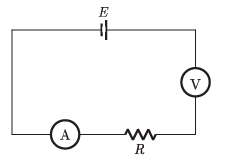Reason : An ideal voltmeter draws almost no current due to very large resistance, and hence (V) and (a) will read zero.

Solution: Here voltmeter is connected in series in the circuit. And it has a high input impedance hence the circuit is behaving like an open circuit. So no current is flowing in the circuit and the ammeter is showing zero. But voltmeter will show the emf of the battery, i.e. E.
QUESTION: 6

Directions: In the following questions, a statement of assertion (A) is followed by a statement of reason (R). Mark the correct choice as:

Assertion : The resistivity of the conductor increases with the increasing temperature.

Reason : The resistivity is the reciprocal of the conductivity.

Solution: The resistivity of the conductors is directly proportional to temperature.
QUESTION: 7

Directions: In the following questions, a statement of assertion (A) is followed by a statement of reason (R). Mark the correct choice as:

Assertion : Resistance of 50 W bulb is greater than that of 100 W.

Reason : Resistance of bulb is inversely proportional to rated power.

Solution: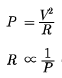(Same rated voltage)

QUESTION: 8

Directions: In the following questions, a statement of assertion (A) is followed by a statement of reason (R). Mark the correct choice as:

Assertion : Two resistance having value R each. Their equivalent resistance is R/2.

Reason : Given Resistance is connected in parallel.

Solution: When two resistance R1 and P2 connected in parallel than their equivalent resistance will be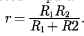QUESTION: 9

Directions: In the following questions, a statement of assertion (A) is followed by a statement of reason (R). Mark the correct choice as:

Assertion : Kirchoff’s rule follows from conservation of charge.

Reason : Kirchoff’s loop rule follows from conservation of momentum.

Solution: Kirchoff’s loop rule follows from conservation of energy.
QUESTION: 10

Directions: In the following questions, a statement of assertion (A) is followed by a statement of reason (R). Mark the correct choice as:

Assertion : Longer wires have greater resistance and the smaller wires have lesser resistance.

Reason : Resistance is inversely proportional to the length of the wire.

Solution:
QUESTION: 11

Directions: In the following questions, a statement of assertion (A) is followed by a statement of reason (R). Mark the correct choice as:

Assertion: The product of resistivity and conductivity of a conductor depends on the material of the conductor.

Reason : Because each resistivity and conductivity depends on the material of the conductor.

Solution:

Conductivity = 1 / Resistivity

Conductivity X resistivity = 1

QUESTION: 12

Directions: In the following questions, a statement of assertion (A) is followed by a statement of reason (R). Mark the correct choice as:

Assertion : When current through a bulb decreases by 0.5% the glow of the bulb decreases by 1%.

Reason : Glow (Power) which is directly proportional to square of current.

Solution: Glow = Power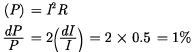QUESTION: 13

Directions: In the following questions, a statement of assertion (A) is followed by a statement of reason (R). Mark the correct choice as:

Assertion : 40 W tube light gives more light in comparison to 40 w bulb.

Reason : Light produced is the same from the same power.

Solution: In tube light the majority portion of radiation comes under visible region while bulb radiation consists of visible, ultraviolet, infrared radiation giving less visible part.
QUESTION: 14

Directions: In the following questions, a statement of assertion (A) is followed by a statement of reason (R). Mark the correct choice as:

Assertion : In a simple battery circuit the point of lowest potential is the positive terminal of the battery.

Reason : The current flows towards the point of the lower potential as it flows in such a circuit from the negative to the positive terminal.

Solution:

It is quite clear that in a battery circuit, the point of lowest potential is the negative terminal of the battery and the current flows from higher potential to lower potential.

QUESTION: 15

Directions: In the following questions, a statement of assertion (A) is followed by a statement of reason (R). Mark the correct choice as:

Assertion : The value of the current in the ammeter is the same, independent of its position in the electric circuit.

Reason : In a series combination of resistors the current is the same in every part of the circuit or the same current through each resistor.

Solution:
QUESTION: 16

Directions: In the following questions, a statement of assertion (A) is followed by a statement of reason (R). Mark the correct choice as:

Assertion : A torch bulb gives light if operated on AC of the same voltage and current as DC.

Reason : Heating effect is common to both AC and DC.

Solution: The bulbs are filled with chemically inactive gases. Nitrogen and oxygen gases are inactive and are filled in order to prolong the life of filament The torch bulbs are operated on heating effect if same voltage DC or same AC rms voltage is applied produces the same heating effect irrespective of the direction of current.
QUESTION: 17

Directions: In the following questions, a statement of assertion (A) is followed by a statement of reason (R). Mark the correct choice as:

Assertion : A resistor of resistance R is connected to an ideal battery. If the value of R is decreased, the power dissipated in the circuit will increase.

Reason : The power dissipated in the circuit is directly proportional to the resistance of the circuit.

Solution: Here,

P =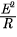so P ∝ R only when I is constant.

Here I increase as R is decreased. Hence the reason is wrong.

QUESTION: 18

Directions: In the following questions, a statement of assertion (A) is followed by a statement of reason (R). Mark the correct choice as:

Assertion : Resistivity of material may-change with temperature.

Reason : Resistivity is a material property & independent of temperature.

Solution: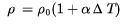The equation shows that resistivity varies with temperature.

QUESTION: 19

Directions: In the following questions, a statement of assertion (A) is followed by a statement of reason (R). Mark the correct choice as:

Assertion : Copper is used to make electric wires.

Reason : Copper has very low electrical resistance.

Solution: A low electrical resistance of copper makes it a good electric conductor. So, it is used to make electric wires.Use Code STAYHOME200 and get INR 200 additional OFF Use Coupon Code